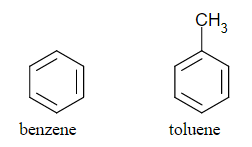# 29 Multicomponent Equilibrium

Learning Objectives

By the end of this section, you should be able to:

Apply properties of ideal mixtures to describe vapour-liquid separations using Raoult’s law and Henry’s law

An ideal mixture contains substances with similar molecular interactions (which usually means similar structures or functional groups). e.g. benzene and toluene, with both being non-polar and of a similar molecular sizeImage from Darkness3560 via Wikimedia Commons/CC0

A combination of two substances is called a binary mixture. For a binary mixture, there is no longer a set temperature and pressure for a boiling point. Instead, there is a range of temperature or pressure values where the mixture is in vapour-liquid equilibrium. These are bounded by two points called the bubble point and dew point. We’ll explore these concepts more thoroughly when looking at diagrams for these binary mixture systems.

## Vapour-Liquid Equilibrium

In a closed container, vapour-liquid equilibrium can be achieved by the same number of molecules vapourizing and condensing at any given period of time (meaning no net change in the system). The temperature and pressure have to be kept constant if we are truly at equilibrium (again, no net change in any intensive variable).

We usually denote:

$T$: overall temperature

$P$: overall pressure

$x_{i}$: the mole fraction of substance $i$ in the liquid phase

$y_{i}$: the mole fraction of substance $i$ in the vapour phase

## Estimating the Molar Fraction of a Component at Vapour-Liquid Equilibrium

### Raoult’s law

Raoult’s law is used when all components are in relatively significant quantities or they are chemically very similar (generally in terms of intermolecular interactions).

$p_{i}=y_{i}×P=x_{i}×p^*_{i}(T)$

$pi^*(T)$ means that the vapour pressure is a function of temperature, which can be calculated using the Antoine Equation (there are other equations, but in this class we will stick to the Antoine equation).

Exercise: Estimating Vapour Pressure for Ethanol

At 298 K, the vapour pressure of water is 3,138 Pa and the vapour pressure of ethanol is 7,817 Pa. Suppose we have a mixture of only water and ethanol in a closed container, at 298 K, what is the mole fraction of ethanol in the vapour phase ($y_{enthaol}$) at equilibrium if the liquid is 50 mol% water and 50 mol% ethanol?

### Solution

Step 1: First note here ethanol and water both have very polar OH groups, so we will consider their intermolecular interactions similar and apply Raoult’s law. Rearrange the Raoult’s law to isolate $y_{i}$:
\begin{align*}
y_{i}×P & =x_{i}×p^*_{i}(T)\\
y_{i} & = \frac{x_{i}×p^*_{i}(T)}{P}
\end{align*}

Here we know both $x_i$, which was given to be 0.5 (50 mol%) for ethanol, and $p^*_{i}$ for ethanol, again given in the question prompt. But now we need to find pressure ($P$).

Step 2: To find $P$ we’ll first apply the ideal gas law to the gas phase in this system. We know that for ideal gases the partial pressures ($p_{i}$) add up to the total system pressure ($P$). We can then use Raoult’s law to express partial pressures in terms of liquid mole fractions and vapour pressures (all of which we know in this case):
\begin{align*}
P & =p_{water}+p_{ethanol}\\
& = x_{water}×p^*_{water}+x_{ethanol}×p^*_{ethanol}\\
& = 0.5×3138Pa + 0.5×7817Pa \\
& = 5477.5 Pa
\end{align*}

Step 3: Subsitute $P$ into the expression for $y_{ethanol}$:
\begin{align*}
y_{ethanol} & = \frac{x_{ethanol}×p^*_{ethanol}(298K)}{P} \\
& =\frac{0.5×7817Pa}{5477.5Pa} \\
& = 0.71
\end{align*}

Finally we could ask ourselves, “does this answer make sense?”. If we look up the normal boiling point temperature of water (100 °C ) and ethanol (78 °C), we see ethanol is more volatile (it boils at a lower temperature). This tells us to expect a greater mole fraction of it in the vapour phase than the liquid phase, which matches what we find with our calculations.

### Henry’s Law

Henry’s law is used to describe the concentration of a gas (or very volatile component) dissolved in a liquid. This is typically used to describe components in a mixture that are near or above their critical temperature (think back to the difference between vapours ($T < T_c$) and gases ($T > T_c$).

$p_{i}=y_{i}×P=\frac{x_{i}}{H_{i}(T)}$

$H_{i}(T)$ Henry’s constant, usually given for a solvent in a given range of temperature.

The Henry’s law constant $H_{i}(T)$ can be given in different units, such as $k\!Pa^{-1}$, $\frac{mol}{L·k\!Pa}$, $\frac{mol}{kg*bar}$, which will change the corresponding unit for $x_{i}$. $x_{i}$ can be used to represent the solubility of the gas in per mole / per volume / per mass of solvent.

Henry’s law is generally more valid when $x_{i}$ is close to 0 (very dilute solutions).

Exercise: Calculating Partial Pressure Using Henry’s Law

$^{}$The concentration of dissolved oxygen in water exposed to gaseous oxygen at a partial pressure of 101.3 kPa at 20 °C is $1.38× 10^{−3}molL^{−1}$. Use Henry’s law to determine the solubility of oxygen when its partial pressure is 20.7 kPa at the same temperature. Note that this approximates the pressure of oxygen in the earth’s atmosphere.

### Solution

Step 1: Calculate Henry’s law constant from data with oxygen pressure at 101.3 kPa
\begin{align*}
p_{i} &=\frac{x_{i}}{H_{i}(T)}\\
H_{i}(T) & =\frac{x_{i}}{p_{i}}\\
& =\frac{1.38×10^{−3}\frac{mol}{L}}{101.3kPa}\\
&= 1.36×10^{−5} \frac{mol}{L·k\!Pa}
\end{align*}

Step 2: Apply Henry’s law another time for an oxygen partial pressure at 20.7 kPa:
\begin{align*}
p_{i} &=\frac{x_{i}}{H_{i}(T)}\\
x_{i} & = p_{i}×H_{i}(T)\\
& =20.7kPa×1.36×10^{−5} \frac{mol}{L·k\!Pa}\\
&= 2.82×10^{−4} \frac{mol}{L}
\end{align*}

## References

 OpenStax Chemistry 2e. 2019 Feb. 11.3 Solubility. [online] <https://openstax.org/books/chemistry-atoms-first-2e/pages/11-3-solubility> [Assessed 18 May 2020]